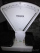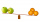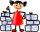# Fraction calculator

The calculator performs basic and advanced operations with fractions, expressions with fractions combined with integers, decimals, and mixed numbers. It also shows detailed step-by-step information about the fraction calculation procedure. Solve problems with two, three, or more fractions and numbers in one expression.

## Result:

### 11/7 * 3/5 = 24/35 ≅ 0.6857143

Spelled result in words is twenty-four thirty-fifths.

### How do you solve fractions step by step?

1. Conversion a mixed number 1 1/7 to a improper fraction: 1 1/7 = 1 1/7 = 1 · 7 + 1/7 = 7 + 1/7 = 8/7

To find a new numerator:
a) Multiply the whole number 1 by the denominator 7. Whole number 1 equally 1 * 7/7 = 7/7
b) Add the answer from previous step 7 to the numerator 1. New numerator is 7 + 1 = 8
c) Write a previous answer (new numerator 8) over the denominator 7.

One and one seventh is eight sevenths
2. Multiple: 8/7 * 3/5 = 8 · 3/7 · 5 = 24/35
Multiply both numerators and denominators. Result fraction keep to lowest possible denominator GCD(24, 35) = 1. In the following intermediate step, the fraction result cannot be further simplified by canceling.
In other words - eight sevenths multiplied by three fifths = twenty-four thirty-fifths.

#### Rules for expressions with fractions:

Fractions - use the slash “/” between the numerator and denominator, i.e., for five-hundredths, enter 5/100. If you are using mixed numbers, be sure to leave a single space between the whole and fraction part.
The slash separates the numerator (number above a fraction line) and denominator (number below).

Mixed numerals (mixed fractions or mixed numbers) write as non-zero integer separated by one space and fraction i.e., 1 2/3 (having the same sign). An example of a negative mixed fraction: -5 1/2.
Because slash is both signs for fraction line and division, we recommended use colon (:) as the operator of division fractions i.e., 1/2 : 3.

Decimals (decimal numbers) enter with a decimal point . and they are automatically converted to fractions - i.e. 1.45.

The colon : and slash / is the symbol of division. Can be used to divide mixed numbers 1 2/3 : 4 3/8 or can be used for write complex fractions i.e. 1/2 : 1/3.
An asterisk * or × is the symbol for multiplication.
Plus + is addition, minus sign - is subtraction and ()[] is mathematical parentheses.
The exponentiation/power symbol is ^ - for example: (7/8-4/5)^2 = (7/8-4/5)2

#### Examples:

subtracting fractions: 2/3 - 1/2
multiplying fractions: 7/8 * 3/9
dividing Fractions: 1/2 : 3/4
exponentiation of fraction: 3/5^3
fractional exponents: 16 ^ 1/2
adding fractions and mixed numbers: 8/5 + 6 2/7
dividing integer and fraction: 5 ÷ 1/2
complex fractions: 5/8 : 2 2/3
decimal to fraction: 0.625
Fraction to Decimal: 1/4
Fraction to Percent: 1/8 %
comparing fractions: 1/4 2/3
multiplying a fraction by a whole number: 6 * 3/4
square root of a fraction: sqrt(1/16)
reducing or simplifying the fraction (simplification) - dividing the numerator and denominator of a fraction by the same non-zero number - equivalent fraction: 4/22
expression with brackets: 1/3 * (1/2 - 3 3/8)
compound fraction: 3/4 of 5/7
fractions multiple: 2/3 of 3/5
divide to find the quotient: 3/5 ÷ 2/3

The calculator follows well-known rules for order of operations. The most common mnemonics for remembering this order of operations are:
PEMDAS - Parentheses, Exponents, Multiplication, Division, Addition, Subtraction.
BEDMAS - Brackets, Exponents, Division, Multiplication, Addition, Subtraction
BODMAS - Brackets, Of or Order, Division, Multiplication, Addition, Subtraction.
GEMDAS - Grouping Symbols - brackets (){}, Exponents, Multiplication, Division, Addition, Subtraction.
Be careful, always do multiplication and division before addition and subtraction. Some operators (+ and -) and (* and /) has the same priority and then must evaluate from left to right.

## Fractions in word problems:

• SayavongSayavong is making cookies for the class. He has a recipe that calls for 3 and 1/2 cups of flour. He has 7/8 of a cup of wheat flour, and 2 and 1/2 cups of white flour. Does Mr. Sayavong have enough flour to make the cookies?
• Ten fractionsWrite ten fractions between 1/3 and 2/3
• A smallA small book took one-sixth of a ream of paper to make. The team said they could make nine books from 3 whole reams of paper. Are they correct?
• Comparing and sortingArrange in descending order this fractions: 2/7, 7/10 & 1/2
• SandySandy, John and Marg baked pies for the Bake Sale. Sandy cut his pies into 6ths, John but his into 8ths and Marg cut hers into quarters. Sandy sold 11/6, John sold  1 3/8 pies and Marg sold 9/4 pies. Who sold the most pies? Who sold the fewest?
• Sort fractionsWhich of the following fractions is the largest? 29/36 5/6 7/9 3/4
• CompareCompare fractions (34)/(3) and (12)/(4). Which fraction of the lower?
• How manyHow many integers are greater than 547/3 and less than 931/4?
• Turtles 2A box turtle hibernates in the sand at 11 5/8. A spotted turtle hibernates at 11 16/25 feet. Which turtle is deeper? Write answer as number 1 or 2.
• Paper collectingAt the paper collecting contest gathered Franta 2/9 ton, Karel 1/4 ton, and Patrick 19/36 tons of paper. Who has gathered the most and the least?
• Engineer KažimírThe difference between politicians-demagogues and reasonable person with at least primary education beautifully illustrated by the TV show example. "Engineer" Kažimír says that during their tenure there was a large decline in the price of natural gas, pri
• Sort fractionsWhich is larger 3/7, 3/8, 3/9, 3/6 =
• ArrangeArrange the following in descending order: 0.32, 2on5, 27%, 1 on 3